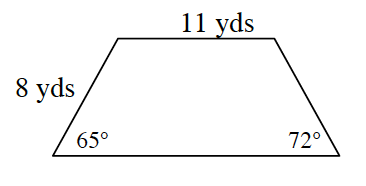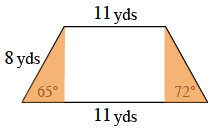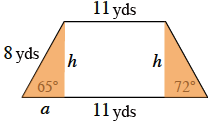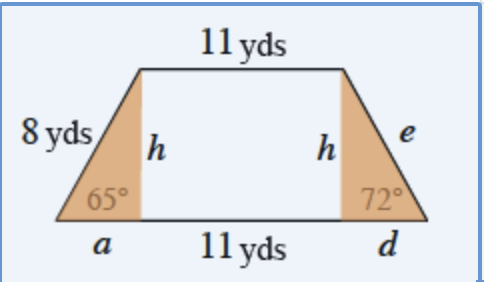Home > CCG > Chapter 6 > Lesson 6.2.3 > Problem6-76

6-76.

Find the area and perimeter of the trapezoid at right.Separate the trapezoid into $3$ separate figures: a right triangle at left, a rectangle in the middle, and a right triangle at right.

Vertical segments drawn from top vertices, perpendicular to the bottom base, creating shaded right triangles on right & left of trapezoid.

Label the height of each triangle, $h$. For the left triangle, label the second leg, $a$. Solve for $h$ and $a$.

Labels added to each vertical segment, h, and base of left shaded triangle, a.

$\sin(65º)=\frac{h}{8}$ and $\cos(65º)=\frac{a}{8}$

Follow a similar process for the second triangle.

Labels added to right shaded triangle, e on the hypotenuse, d on the base.

Area $\approx100.55$ yds$^2$
Perimeter $\approx43.36$ yds